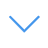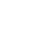# Animals Math Puzzle

Animals Math Puzzles is one of the Math Games that you can play on UGameZone.com for free. Animals Math Puzzles is mathematical game with goal to solve 50 different mathematical problems all in limited time per task. Tasks are created as linear equations with 2 and 3 variables.

Read Moreاضغط على 【Esc】 للخروج من وضع ملء الشاشة

# Animals Math Puzzle

Animals Math Puzzles is one of the Math Games that you can play on UGameZone.com for free. Animals Math Puzzles is mathematical game with goal to solve 50 different mathematical problems all in limited time per task. Tasks are created as linear equations with 2 and 3 variables.لتشغيل اللعبة ، تحتاج إلى السماح بتشغيل Adobe Flash Player في متصفحك.

You can use UGameZone Flash Enabler plugin to enable Flash automatically. Or you can follow our instructions to enable Flash manually.

play automatically

## Game Ratings

89%

يلعب 1760

تقييم 89%

الأصوات 734

734
68
Category
Math Games
الكلمات
وصف

Animals Math Puzzles is one of the Math Games that you can play on UGameZone.com for free. Animals Math Puzzles is mathematical game with goal to solve 50 different mathematical problems all in limited time per task. Tasks are created as linear equations with 2 and 3 variables.

Step 1
Step 2